Courses

# Olympiad Test: Alphabet Test - 2

## 20 Questions MCQ Test Mathematics Olympiad Class 7 | Olympiad Test: Alphabet Test - 2

Description
This mock test of Olympiad Test: Alphabet Test - 2 for Class 7 helps you for every Class 7 entrance exam. This contains 20 Multiple Choice Questions for Class 7 Olympiad Test: Alphabet Test - 2 (mcq) to study with solutions a complete question bank. The solved questions answers in this Olympiad Test: Alphabet Test - 2 quiz give you a good mix of easy questions and tough questions. Class 7 students definitely take this Olympiad Test: Alphabet Test - 2 exercise for a better result in the exam. You can find other Olympiad Test: Alphabet Test - 2 extra questions, long questions & short questions for Class 7 on EduRev as well by searching above.
QUESTION: 1

### Direction: In each of the following questions find out how many such pairs of letters are there in the given word each of which has as many letters between them in the word as in the English alphabet. PARADISE

Solution:

(b) PARARADP Q R A B C D

QUESTION: 2

### Direction: In each of the following questions find out how many such pairs of letters are there in the given word each of which has as many letters between them in the word as in the English alphabet. HORIZON

Solution: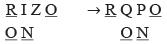QUESTION: 3

### Direction: In each of the following questions find out how many such pairs of letters are there in the given word each of which has as many letters between them in the word as in the English alphabet. LANGUISH

Solution: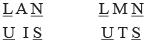QUESTION: 4

Direction: In each of the following questions find out how many such pairs of letters are there in the given word each of which has as many letters between them in the word as in the English alphabet.
Which letter in word CYBERNETICS occupies the same position as it does in the English alphabet?

Solution:

I is the 8th letter in the word as well as in the English alphabet.

QUESTION: 5

Direction: In each of the following questions find out how many such pairs of letters are there in the given word each of which has as many letters between them in the word as in the English alphabet.
HACKLE

Solution:

H A C K H I J K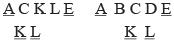QUESTION: 6

Direction: In each of the following questions, find out which of the letter – series follows the given rule.
Number of letters skipped in between adjacent letters in the series is two.

Solution:QUESTION: 7

Direction: In each of the following questions, find out which of the letter – series follows the given rule.
Q. Number of letters skipped in between the adjacent letters in the series are consecutive even numbers.

Solution: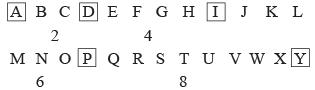2, 4, 6, 8 are consecutive even numbers.

QUESTION: 8

Direction: In each of the following questions, find out which of the letter – series follows the given rule.
Q. Number of letters skipped in between adjacent letters of the series starting from behind increase by one.

Solution: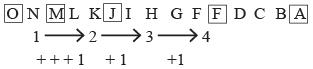QUESTION: 9

Number of letters skipped in between adjacent letters in the series decrease by two

Solution: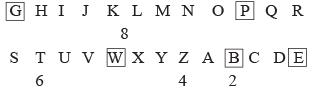QUESTION: 10

Direction: In each of the following questions, find out which of the letter – series follows the given rule.
Q. Number of letters skipped in between adjacent letters in the series doubles very lime.

Solution: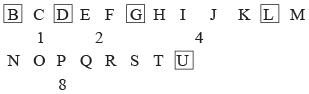QUESTION: 11

Direction: In each of the following questions, find out which of the letter – series follows the given rule.
Q. In the following series, the number of letters skipped in between the adjacent letters are in ascending order, i.e., 1, 2, 2, 3 which one of the following letter groups does not obey this rule?

Solution: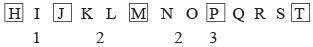QUESTION: 12

Direction: In each of the following questions, find out which of the letter – series follows the given rule.
Q. Number of letters skipped in between adjacent letters in the series is in the order of 2, 5, 7, 10.

Solution: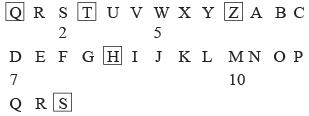QUESTION: 13

Direction: In each of the following questions, find out which of the letter – series follows the given rule.
Q. The letters are not according to a general rule.

Solution: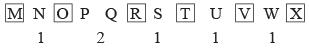QUESTION: 14

Direction: In each of the following questions, find out which of the letter – series follows the given rule.
Number of letters skipped in between adjacent letters in the series is odd.

Solution: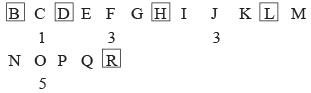QUESTION: 15

Direction: In each of the following questions, find out which of the letter – series follows the given rule.
Number of letters skipped in between adjacent letters in the series increases by one.

Solution: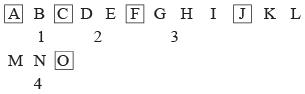QUESTION: 16

Direction: Study the following arrangement of the english alphabet and answer the questions given below:​
Q. Which of the following pairs of letters has as many letters between them in the above arrangement as there are between them in the english alphabet?

Solution:

M and O have just one letter between them in the given series as well as in the english alphabet.

QUESTION: 17

Direction: Study the following arrangement of the english alphabet and answer the questions given below:​
Q. How many T’s are there in the following sequence which are immediately preceded by P but not immediately followed by S?
S T P Q T S P T R P T S T P S T Q P T R P T M P T S

Solution: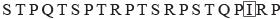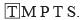QUESTION: 18

Direction: Study the following arrangement of the english alphabet and answer the questions given below:​
Q. How many L’s are there which do not have R preceding them and also do not have T following them? Z Q S T L R M N Q N R T U V X R L T A S L T Q R S L T

Solution: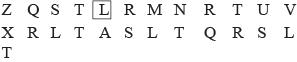QUESTION: 19

Direction: Each of following questions is based on the following alphabet series.
Q. If 1st and 26th, 2nd and 25th, 3rd and 24th, and so on, letters of the english alphabet are paired, then which of the following pairs is correct?

Solution:

The pairing up of letters may be done as shown.
AZ, BY, CX, DW, EV, FU, GT, HS, IR, JQ, KP, LO, MN.

QUESTION: 20

Direction: Each of following questions is based on the following alphabet series.
Q. Which letter should be ninth letter to the left of ninth letter from the right, if the first half of the given alphabet is reversed?

Solution:

M L K J I H G F E D C B  W.
O P Q R S T U V W X Y Z. the 9th letter from the right is R.